# Nickzom Calculator Calculates and Solves for Polynomials | Cubic and Quartic

According to Wikipedia,

In mathematics, a polynomial is an expression consisting of variables (also called indeterminates) and coefficients, that involves only the operations of additionsubtractionmultiplication, and non-negative integer exponents of variables.

Nickzom Calculator is the very first calculator encyclopedia to come out from Nigeria. It is capable of solving over 50,000 calculations and presenting the steps (workings). Fortunately, Nickzom Calculator can compute conception and best intercourse dates.

You can access Nickzom Calculator via any of these means:
Web – https://www.nickzom.org/calculator-plus
Apple (Paid) – https://itunes.apple.com/us/app/nickzom-calculator/id1331162702?mt=8

Once, you have access to Nickzom Calculator proceed to the Calculator Map, click on Polynomials under Mathematics sectionFor Nickzom Calculator, there are two polynomials which are cubic and quartic.

A cubic equation or polynomial has a degree or order of 3 whereas a quartic equation or polynomial has a degree or order of 4.Find the roots of f(x) = 2x3 + 3x2 – 11x – 6 = 0, given that it has at least one integer root.From the example above, you can see that the coefficient of x3 is 2, the coefficient of x2 is 3, the coefficient of x is -11, the constant before the equality sign is -6, the constant after the equality sign is 0.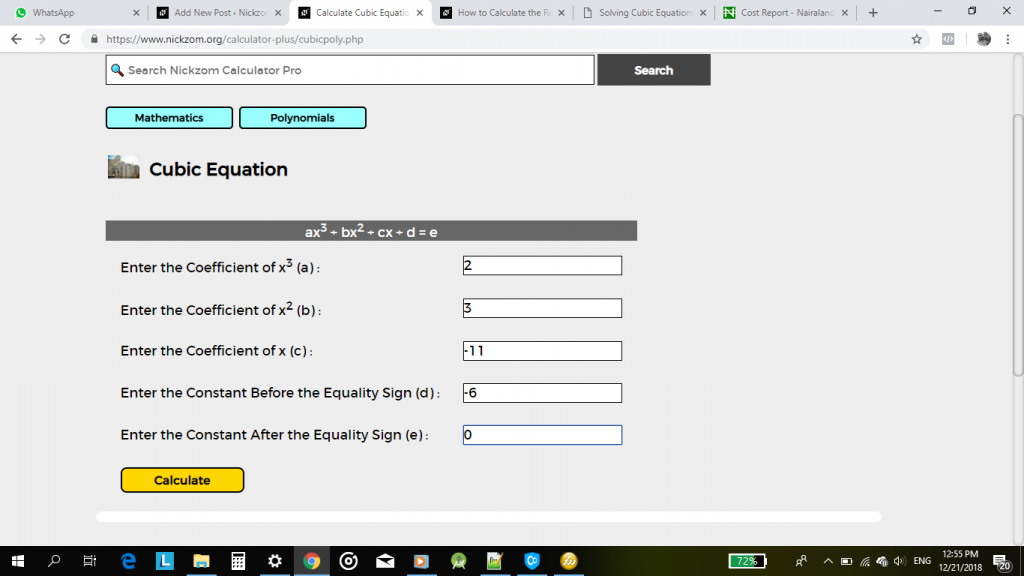Now, click on Calculate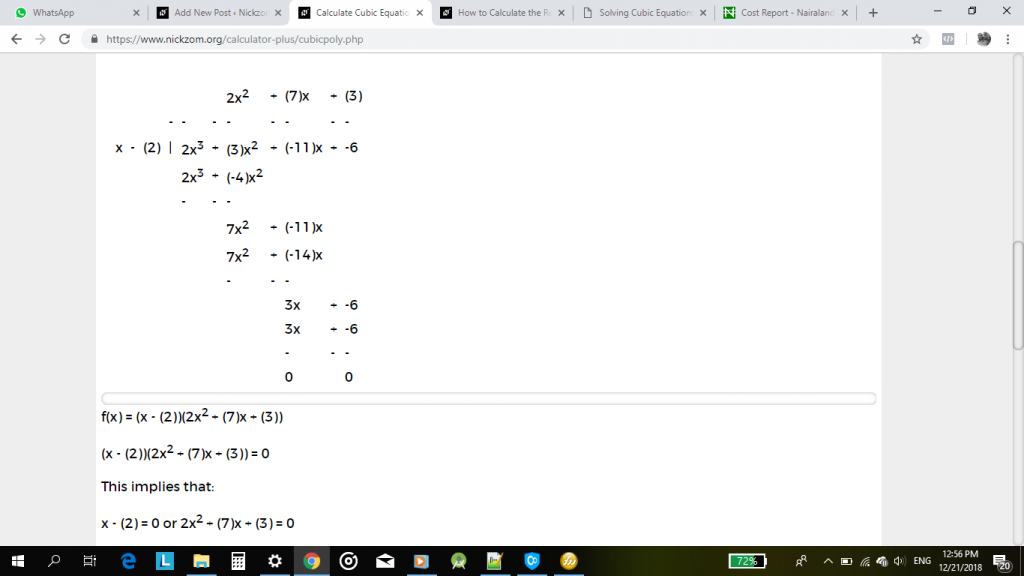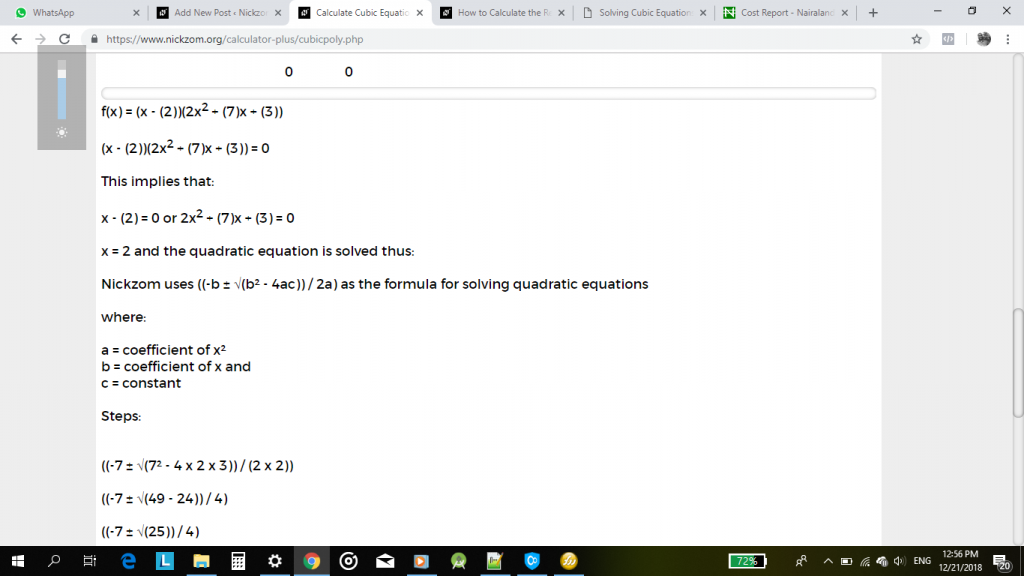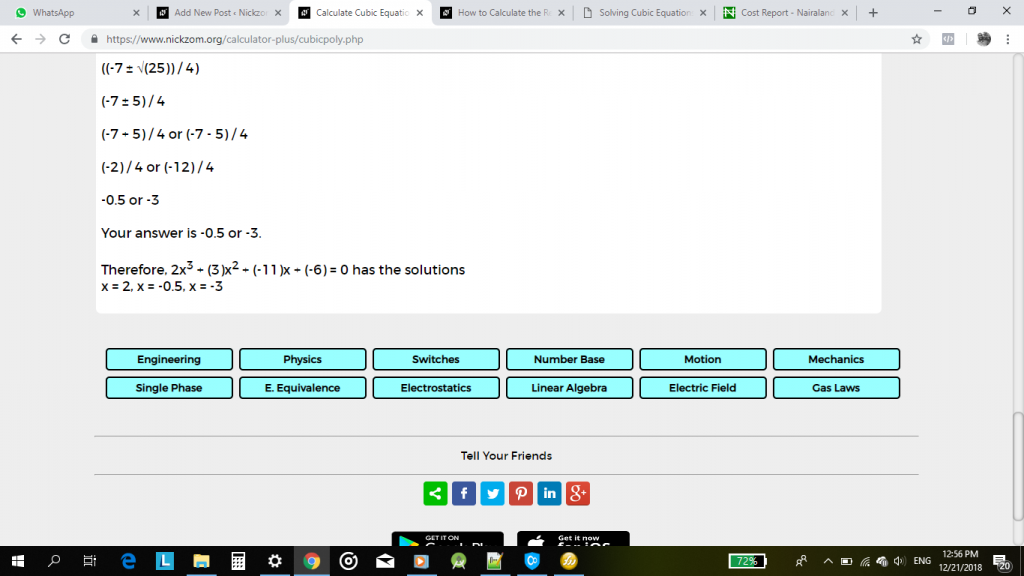From Nickzom Calculator – The Calculator Encyclopedia, the values of x are: 2, -0.5 and -3.

Now, let me show you how to use Nickzom Calculator – The Calculator Encyclopedia to get answers for a quartic equation.Find the roots of f(x) = 3x4   + 6x3   – 123x2   – 126x + 1080 = 0, given that it has 4 real roots.From the example above, you can see that the coefficient of x4 is 3, the coefficient of x3 is 6, the coefficient of x2 is -123, the coefficient of x is -126, the constant before the equality sign is 1080 and the constant after the equality sign is 0.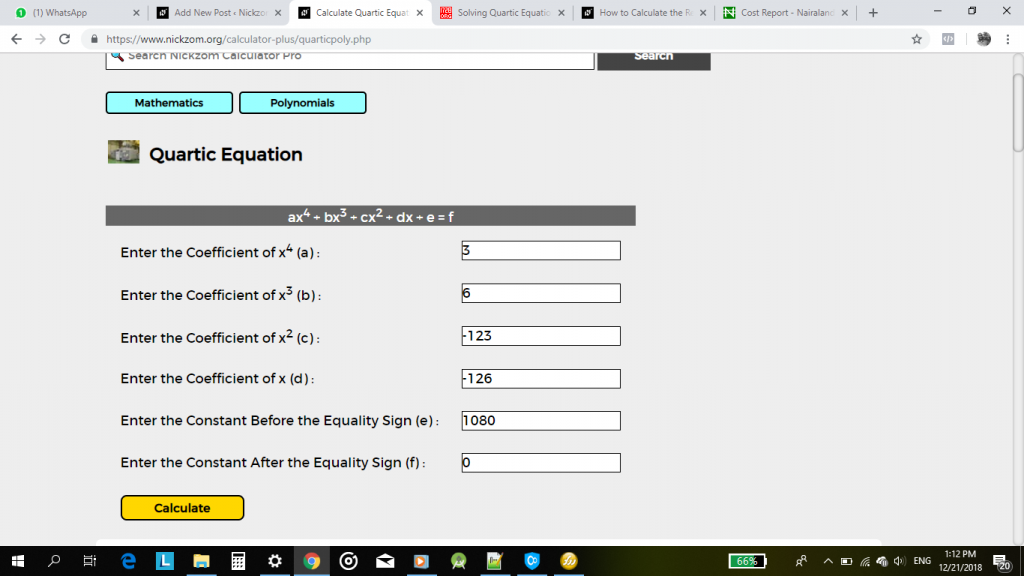Now, click on Calculate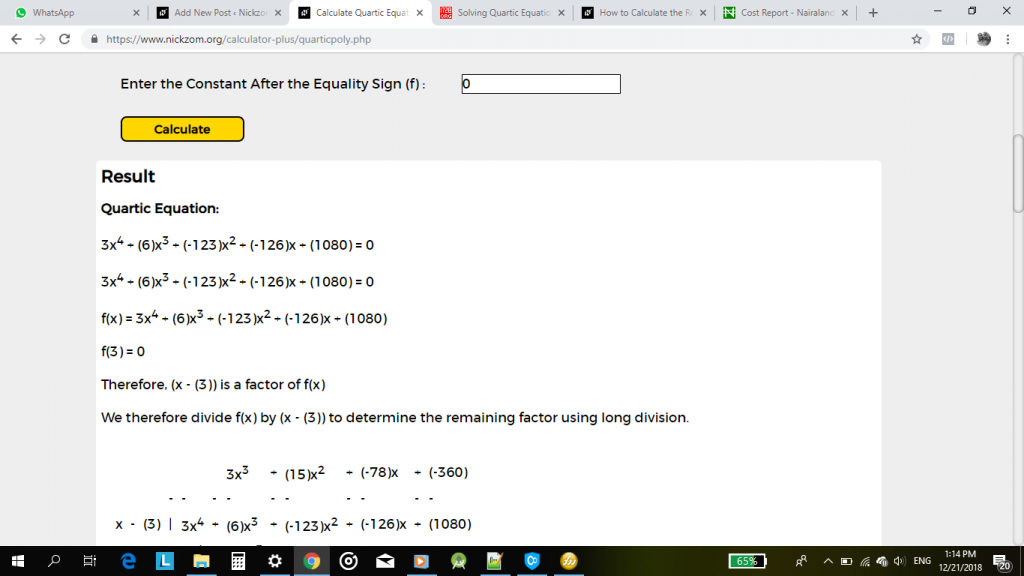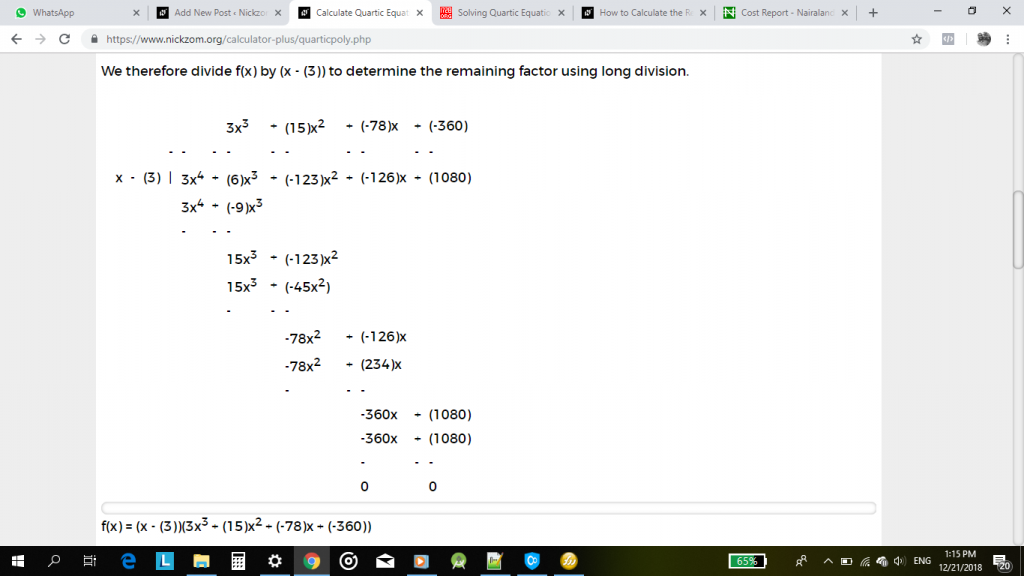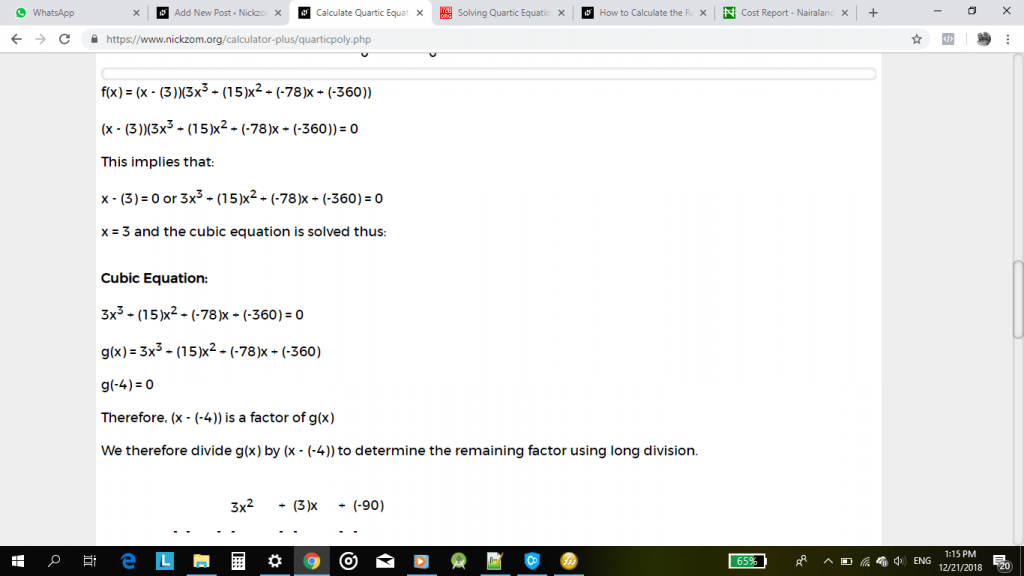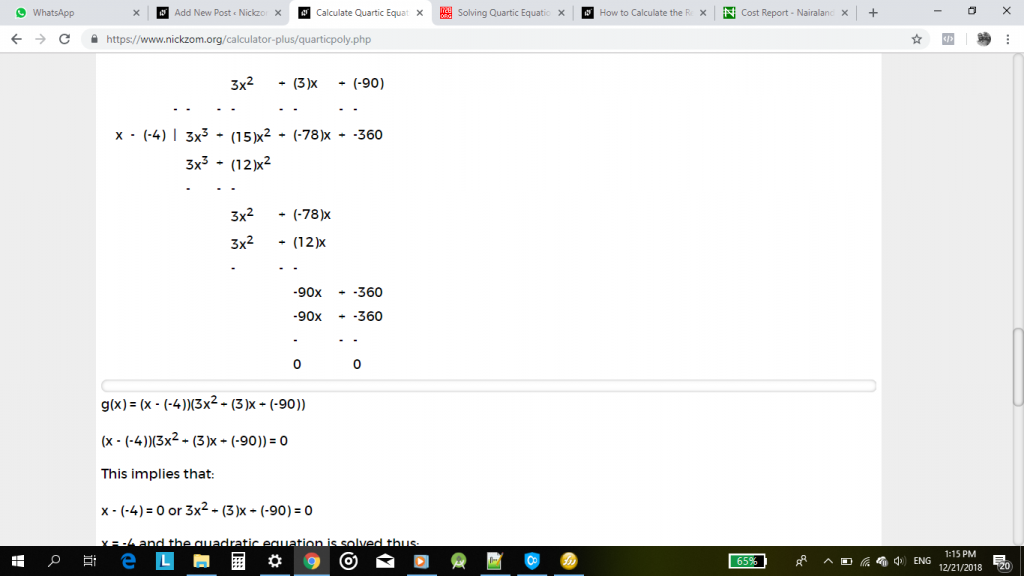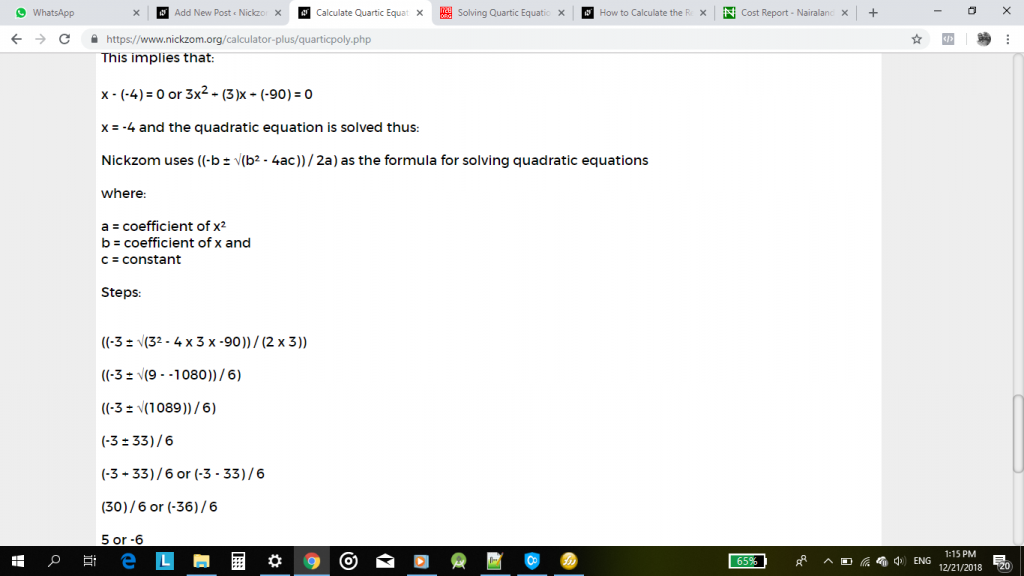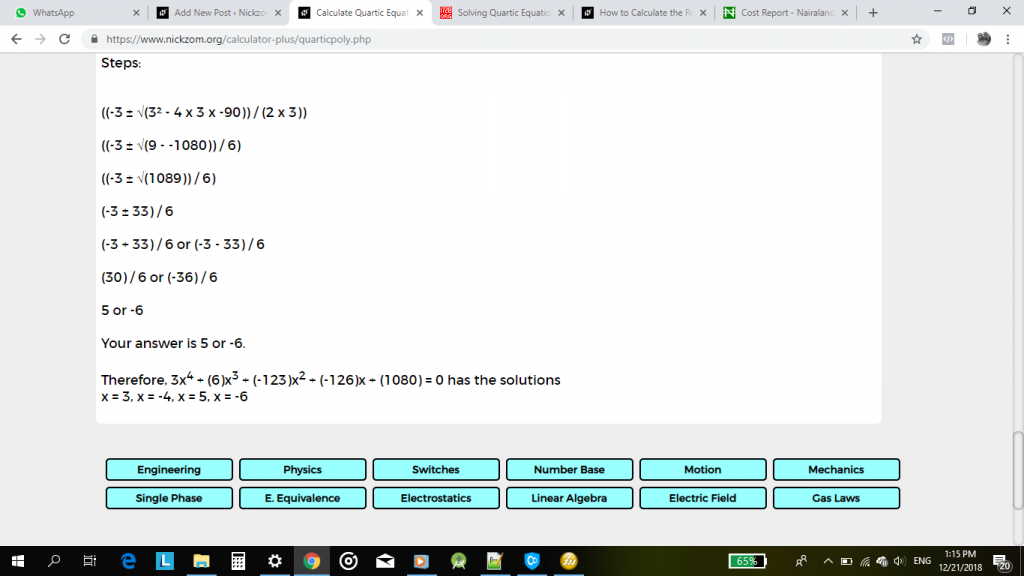From Nickzom Calculator – The Calculator Encyclopedia, the values of x are: 3, -4, 5, -6.